﻿ 基于网购忠诚度的性别差异分析

# 基于网购忠诚度的性别差异分析Analysis of Gender Based on Online Shopping Loyalty

Abstract: In the E-market environment, consumers have more options and more free when they choose something. It is particularly important for the business to improve consumer loyalty. Therefore, the business should know how to recognize their own customers so as to improve customers’ loyalty and gain more profits when they are in the B2C market. In this paper, a non-parametric test—run test, is adopted to test the gender differences of consumers, and analyze whether the gender will impact consumers’ loyalty, so that business can take gender into account when launching appropriate sales strategies to attract customers and achieve greater profits.

1. 引言

2. 研究现状

3. 模型构建

3.1. 忠诚度量化模型构建

F的四个分量应满足以下条件：

1) 非负性

2) 分量越大，忠诚度越高

Browseij表示i顾客对j店铺的浏览量(简写Bij)

Collectij表示i顾客对j店铺的收藏量(简写Cij)

Purchaseij表示i顾客对j店铺的购买量(简写Pij)

i顾客浏览忠诚度指数Browsei = $\underset{\text{j}}{\sum }{\text{B}}_{\text{ij}}^{2}$ (2)

i顾客加购忠诚度指数Add to basketi = $\underset{\text{j}}{\sum }{\text{A}}_{\text{ij}}^{2}$ (3)

i顾客收藏忠诚度指数Collecti = $\underset{\text{j}}{\sum }{\text{C}}_{\text{ij}}^{2}$ (4)

i顾客购买忠诚度指数Purchasei = $\underset{\text{j}}{\sum }{\text{P}}_{\text{ij}}^{2}$ (5)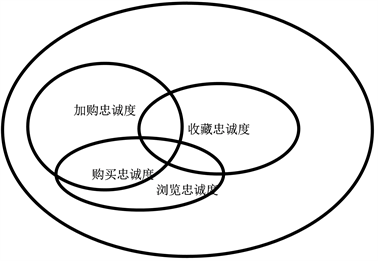Figure 1. Schematic diagram of the relationship between the four indicators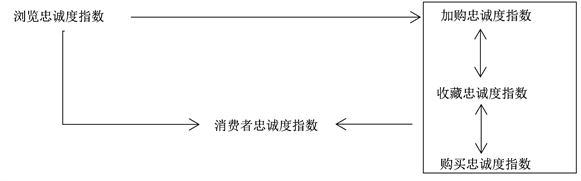Figure 2. Schematic diagram of the relationship between the loyalty indexes

3.2. 数据介绍以及模型分析

Table 2. Example table of consumer visits and browsing loyalty index for different merchants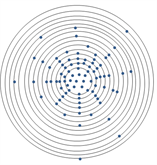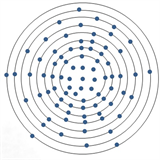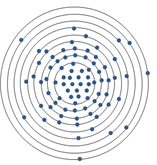*157073消费者 *159731消费者 *204013消费者 *156106消费者 忠诚度指数685 忠诚度指数896 忠诚度指数992 忠诚度指数1176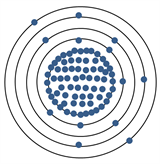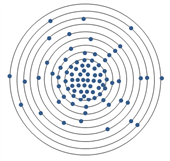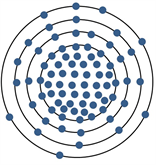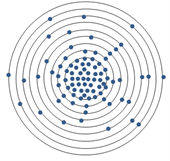*100258消费者 *274376消费者 *160139消费者 *234191消费者忠诚度指数2144 忠诚度指数2670 忠诚度指数3320 忠诚度指数4547

Figure 3. Diagram of the concentrative degree that show consumers’ page views to online shops

4. 性别差异性分析

4.1. 游程检验的方法介绍Table 3. The analysis of procedure checking

4.2. 性别差异性计算过程

4.2.1. 选取全部数据进行性别差异性分析

$\text{Er}=\frac{2\text{n}1\text{n}2}{\text{n}1+\text{n}2}+\frac{1}{2}=\frac{2×2158×1130}{2158+1130}+\frac{1}{2}=1483.8$ (6)

$\begin{array}{c}\text{Dr}=\frac{2\text{n}1\text{n}2\left(2\text{n}1\text{n}2-\text{n}1-\text{n}2\right)}{\text{n}1+\text{n}{2}^{2}\left(\text{n}1+\text{n}2-1\right)}\\ =\frac{2×2158×1130\left(2×2158×1130-2158-1130\right)}{2158+{1130}^{2}\left(2158+1130-1\right)}=668.9\end{array}$ (7)

$\text{Zr}=\frac{|\text{r}-{\text{μ}}_{\text{r}}|}{{\text{σ}}_{\text{r}}}=\frac{|1479-1483.8|}{\sqrt{668.9}}=0.18<1.96$ (8)Table 4. Statistical results of difference-analysis to the whole data

4.2.2. 抽取50%的数据做性别差异性分析

4.2.3. 抽取30%的数据做性别差异性分析Table 5. Statistical results of difference-analysis to 50% dataTable 6. Statistical results of difference-analysis to 30% data

4.3. 结论Table 7. The summary results of proposal

 韩经纶, 韦福祥. 顾客满意与顾客忠诚互动关系研究[J]. 南开管理评论, 2001, 4(6): 8-10, 29.

 陆娟, 芦艳. 服务质量与服务忠诚的多维度关系研究[J]. 财贸研究, 2006(6): 80-87.

 顾客忠诚度[EB/OL].
https://baike.so.com/doc/7555106-7829199.html, 2016-10-19.

 狄佩佩. B2C模式下顾客忠诚度影响因素研究[J]. 现代商业, 2017(14): 40-41.

 唐胡鑫. 关于电商用户忠诚度评估方法的研究[J]. 计算机仿真, 2017, 34(1): 348-351.

 邓爱民, 陶宝, 马莹莹. 网络购物顾客忠诚度影响因素的实证研究[J]. 中国管理科学, 2014, 22(6): 94-102.

 李敏, 张玉莹, 于欣雨. 基于聚类分析的电子商务客户忠诚度研究[J]. 哈尔滨商业大学学报(自然科学版), 2017, 33(2): 175-179.

 林国顺, 李向文. 交通运输模拟建模技术[M]. 大连: 大连海事大学出版社, 2004: 63-67.

Top